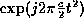Next: Historical Notes Up: The Chirplet Transform: Previous: The Chirplet Transform:

# INTRODUCTION

Underlying a great deal of traditional signal processing theory is the notion of a sinusoidal wave. With the advent of modern computing, and the Fast Fourier transform, the use of and interest in frequency-domain signal processing has increased dramatically. More recently, however, researchers are becoming aware of the limitations of frequency-domain methods. Although the Fourier transform yields perfect reconstruction of a broad class of signals, it does not necessarily provide a meaningful interpretation when the signals lack global stationarity. For example, consider the time series formed by a typical passage of music. An estimate of its power spectrum tells us which musical notes are present (how much energy there is around each of the frequencies), but fails to tell us when each of those notes was sounded.

Much of the recent focus of signal processing is on the so-called time-frequency (TF) methods, which allow us to observe how a spectral estimate evolves over time. One of these TF methods, the short-time Fourier transform (STFT), has been used extensively for analyzing speech, music, and other non-stationary signals. Suppose we want to perform a STFT analysis, but are uncertain what the window size should be. We could perform the STFT of a signal, s(t), using a window of relatively short duration, then stretch the window out a small amount and compute another STFT, and so on, gradually increasing the window size and computing another STFT for each value of window size. Stacking uncountably many of these STFTs on top of one another results in a continuous volumetric representation of s that is a function of time, frequency, and the size of the window (Fig. 1(a)).

Figure 16: FIGURE GOES SOMEWHERE IN THIS GENERAL VICINITY

We will refer to this volumetric representation as the time-frequency-scale (TFS) transform.

Another time-frequency representation (which might more appropriately be called a time-scale representation) is the well-known wavelet transformheil-walnut,mallat:theory,daubechies:transform,strangwavelets. The wavelet transform can be expressed as an inner product of the signal under analysis with a family of translates and dilates of one basic primitive. This primitive is known as the mother wavelet. A member of the wavelet family is produced by a particular one-dimensional affine coordinate transformation acting on the time axis of the mother wavelet; this geometric transformation is parameterized by two numbers (corresponding to the amounts of translation and dilation). The continuous wavelet transform is formed by taking inner products of the signal with the uncountably many members of the two-parameter wavelet family. The continuous wavelet transform is, with an appropriate choice of window/mother-wavelet, simply the time-scale (TS) plane of the TFS volume (Fig. 1(a)).

We begin to see that, even if it is not practical from a computational or data-storage point of view, the time-frequency-scale space is useful from a conceptual point of view. In particular, if we only desire the magnitude TFS volume, we can easily extract this information from the Wigner distribution, by the appropriate coordinate transformations and uniform smoothing of the coordinate-transformed Wigner distributions. A continuous transition from the magnitude TF plane (spectrogram) to the magnitude TS plane (scalogram) is possible through appropriate smoothing of the Wigner distributionrioulgeneralclass.

Now suppose we were to multiply the signal, s(t), by a linear FM (chirp) signaland then compute its STFT. If we vary the hirp rate, c, continuously, and repeat the process uncountably many times, stacking the resulting STFTs one above the other, we obtain a different three-dimensional volume (Fig. 1(b)). This time we have a function of time, frequency and chirprate.

Of course there is no reason to limit ourselves to a choice between these two parameter spaces; to motivate what follows, it will prove helpful to keep in mind a continuous four-dimensional ``time-frequency-scale-chirprate'' (TFSC) parameter space.Next: Historical Notes Up: The Chirplet Transform: Previous: The Chirplet Transform:

Steve Mann
Thu Jan 8 19:50:27 EST 1998